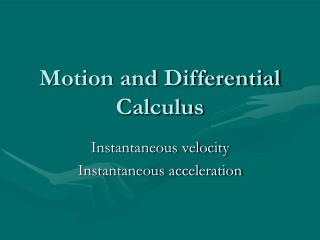DownloadDownload PresentationMotion and Differential Calculus

# Motion and Differential Calculus

Download Presentation## Motion and Differential Calculus

- - - - - - - - - - - - - - - - - - - - - - - - - - - E N D - - - - - - - - - - - - - - - - - - - - - - - - - - -
##### Presentation Transcript

1. Motion and Differential Calculus Instantaneous velocity Instantaneous acceleration

2. Motion and Differential Calculus In preparing for class today I spent a total of ____ hours on reading, homework, and the Student Workbook. • Zero • ½ an hour • 1 ½ hours • 2 hours • 2 ½ hours • 3 hours • Over 3 hours

3. Motion and Differential Calculus The slope at a point on a position-versus-time graph of an object is 1) the object’s speed at that point. 2) the object’s average velocity at that point. 3) the object’s instantaneous velocity at that point. 4) the object’s acceleration at that point. 5) the distance traveled by the object to that point.

4. Motion and Differential Calculus The slope at a point on a position-versus-time graph of an object is 1) the object’s speed at that point. 2) the object’s average velocity at that point. 3) the object’s instantaneous velocity at that point. 4) the object’s acceleration at that point. 5) the distance traveled by the object to that point.

5. Motion and Differential Calculus

6. Motion and Differential Calculus

7. Motion and Differential Calculus

8. Motion and Differential Calculus So far we have been talking about average velocity. What about the velocity at an instant, instantaneous velocity.

9. In calculus you defined We want to defined Motion and Differential Calculus

10. Motion and Differential Calculus The concept of a differential is all in the slope of the tangent line at a point (time).

11. Motion and Differential Calculus Which graph is for an object slowing down and coming to a stop? Which graph is for an object moving with a constant velocity? Which graph is for an object moving with a constantly increasing velocity? Which graph is for an object slowing down and then speeding up in the other direction?

12. Motion and Differential Calculus Which graph is for an object moving at a constant velocity, then acceleration, and finally moving with a constant velocity? Which graph is for an object moving with a constant acceleration? Which graph is for an object moving with a constantly increasing acceleration? Which graph is for an object that starts out accelerating and then moves with a constant velocity?

13. Motion and Differential Calculus

14. Motion and Differential Calculus How is v(t) related to x(t)? How is a(t) related to v(t)?

15. Motion and Differential Calculus Rank in order, from largest to smallest, the accelerations aA–aC at points A – C. 1) aA > aB > aC 2) aC > aA > aB 3) aC > aB > aA 4)aB > aA > aC

16. Motion and Differential Calculus Rank in order, from largest to smallest, the accelerations aA–aC at points A – C. 1) aA > aB > aC 2) aC > aA > aB 3) aC > aB > aA 4)aB > aA > aC

17. (1) (2) (3) (4) Motion and Differential Calculus Which velocity-versus-time graph goes with this position-versus-time graph on the left?

18. (1) (2) (3) (4) Motion and Differential Calculus Which velocity-versus-time graph goes with this position-versus-time graph on the left?

19. Motion and Differential Calculus

20. Motion and Differential Calculus

21. Motion and Differential Calculus

22. Motion and Differential Calculus